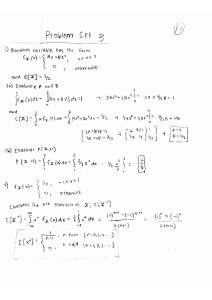# Homework Assignment [Engineering Probability & Statistics]

Make it to the Right and Larger Audience

Paper/Doc

# Homework Assignment [Engineering Probability & Statistics]

A homework assignment from my undergraduate engineering probability and statistics course. Covers expected value, probability density function, and characteristic function.Electrical Engineer
Author brief is empty
Groups:

Tags: